Courses

# Test: Sinusoidal Steady State Analysis

## 10 Questions MCQ Test Electronic Devices | Test: Sinusoidal Steady State Analysis

Description
This mock test of Test: Sinusoidal Steady State Analysis for Electrical Engineering (EE) helps you for every Electrical Engineering (EE) entrance exam. This contains 10 Multiple Choice Questions for Electrical Engineering (EE) Test: Sinusoidal Steady State Analysis (mcq) to study with solutions a complete question bank. The solved questions answers in this Test: Sinusoidal Steady State Analysis quiz give you a good mix of easy questions and tough questions. Electrical Engineering (EE) students definitely take this Test: Sinusoidal Steady State Analysis exercise for a better result in the exam. You can find other Test: Sinusoidal Steady State Analysis extra questions, long questions & short questions for Electrical Engineering (EE) on EduRev as well by searching above.
QUESTION: 1

### i(t) = ?​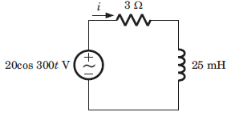Solution: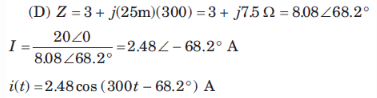QUESTION: 2

### Vc(t) = ?​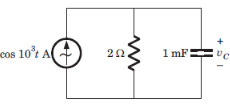Solution: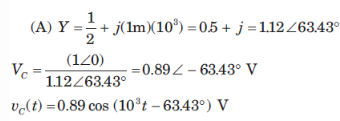QUESTION: 3

### Vc(t) = ?​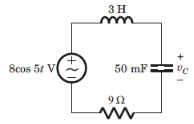Solution: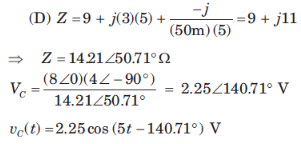QUESTION: 4

i(t) = ?​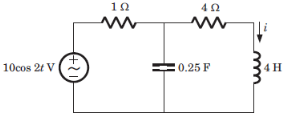Solution: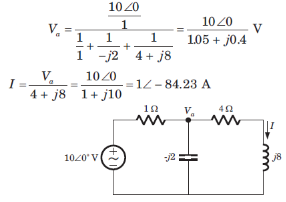QUESTION: 5

In the bridge shown, Z1 = 300 ohm, Z2 = 400 – j300 ohm, Z3 = 200 + j100 ohm. The Z4 at balance is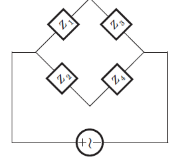Solution:

Use Z1 x Z4 = Z2 x Z3.

QUESTION: 6

Circuit for Q.6 and Q.7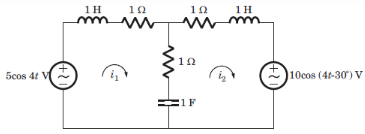Q. ​i1(t) = ?

Solution:
QUESTION: 7

i2(t) = ?

Solution: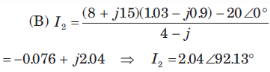QUESTION: 8

In a two element series circuit, the applied voltage and the resulting current are v(t) = 60 + 66 sin (1000t) V, i(t) = 2.3sin (1000t + 68.3) 3 A. The nature of the elements would be

Solution:

RC circuit causes a positive shift in the circuit.

QUESTION: 9

P = 269 W, Q = 150 VAR (capacitive). The power in the complex form is

Solution:

S = P – jQ.

QUESTION: 10

Q = 2000 VAR, pf = 0.9 (leading). The power in complex form is

Solution:

Use cos T = 0.9 or T = 25.84 degrees.
Q = S sin T or S = 4588.6 VA
p = S cos T or P = 0.9 X 4588.6 4129.8 VA.